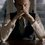# Binomial Coefficient Challenge 6!

Prove the following -

\displaystyle \begin{equation*} 1 - \cfrac{1}{n+\cfrac{2n}{n-3+\cfrac{3(n-1)}{n-5+\cfrac{4(n-2)}{\ddots \cfrac{(m-1)(n-m+3)}{n-(2m-3)}}}}} \end{equation*} = \sum_{0\le k <m}{\dfrac{{(-1)}^k}{\binom{n}{k}}} = \dfrac{n+1}{n+2}\left(1 + \frac{{(-1)}^{m+1}}{\binom{n+1}{m}}\right)

The continued fraction part is optional. It is just a bonus.

This is completely original.Note by Kartik Sharma
3 years, 4 months ago

This discussion board is a place to discuss our Daily Challenges and the math and science related to those challenges. Explanations are more than just a solution — they should explain the steps and thinking strategies that you used to obtain the solution. Comments should further the discussion of math and science.

When posting on Brilliant:

• Use the emojis to react to an explanation, whether you're congratulating a job well done , or just really confused .
• Ask specific questions about the challenge or the steps in somebody's explanation. Well-posed questions can add a lot to the discussion, but posting "I don't understand!" doesn't help anyone.
• Try to contribute something new to the discussion, whether it is an extension, generalization or other idea related to the challenge.

MarkdownAppears as
*italics* or _italics_ italics
**bold** or __bold__ bold
- bulleted- list
• bulleted
• list
1. numbered2. list
1. numbered
2. list
Note: you must add a full line of space before and after lists for them to show up correctly
paragraph 1paragraph 2

paragraph 1

paragraph 2

[example link](https://brilliant.org)example link
> This is a quote
This is a quote
    # I indented these lines
# 4 spaces, and now they show
# up as a code block.

print "hello world"
# I indented these lines
# 4 spaces, and now they show
# up as a code block.

print "hello world"
MathAppears as
Remember to wrap math in $$ ... $$ or $ ... $ to ensure proper formatting.
2 \times 3 $2 \times 3$
2^{34} $2^{34}$
a_{i-1} $a_{i-1}$
\frac{2}{3} $\frac{2}{3}$
\sqrt{2} $\sqrt{2}$
\sum_{i=1}^3 $\sum_{i=1}^3$
\sin \theta $\sin \theta$
\boxed{123} $\boxed{123}$

Sort by:

$\text{S}=\displaystyle\sum_{r=0}^{m-1}\dfrac{(-1)^r}{\dbinom{n}{r}}$

$=\displaystyle\sum_{r=0}^{m-1}(-1)^r\dfrac{r!(n-r)!}{n!}$

$=\displaystyle\sum_{r=0}^{m-1}(-1)^r \dfrac{r!(n-r)!\color{#3D99F6}{(n-r+1+r+1)}}{n!}\color{#D61F06}{\times\dfrac{1}{(n+2)}}$

$=\displaystyle\sum_{r=0}^{m-1}\dfrac{1}{n!(n+2)}\left[(-1)^r\left\{r!(n-r+1)!+(n-r)!(r+1)!\right\}\right]$

$=\displaystyle\sum_{r=0}^{m-1}\dfrac{1}{n!(n+2)}\left[T_{r} - T_{r+1}\right]$

where $T_{r} = (-1)^r r!(n-r+1)!$

Evaluating using telescoping the sum, we have,

$\text{S} = \dfrac{n+1}{n+2}\left(1+\dfrac{(-1)^{m+1}}{\dbinom{n+1}{m}}\right)$

Some other methods can be to use Beta functions or using the result (from Partial Fractions)

$\dfrac{1}{\dbinom{n}{k}}=\sum_{j=1}^k(-1)^{k-j}\binom{k}{j}\dfrac{j}{n-j+1}$

The continued fraction result is fascinating! I'll work on it when I get some time.

- 3 years, 4 months ago

@Kartik Sharma Btw, what was your approach to this problem?

- 3 years, 4 months ago

I used Finite Calculus(Gosper's Method). I thought of beta functions but it was tedious. Telescoping serious was very nice although a bit too tricky.

I will add my method later. For the continued fraction part if you are asking, then I used a standard result in the theory of evaluating continued fractions.

- 3 years, 4 months ago

I was talking about the summation. I assume this Gosper's method?

I have added some explanation in the telescoping part for clarity

- 3 years, 4 months ago

Yes. It is quite general.

- 3 years, 4 months ago